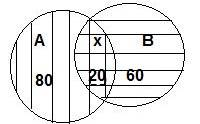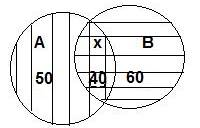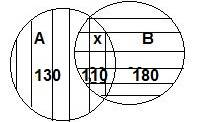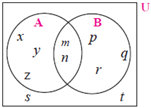SSC BOARD PAPERS IMPORTANT TOPICS COVERED FOR BOARD EXAM 2024

### Practice Set 1.4 Sets Class 9th Mathematics Part I MHB Solution

###### Practice Set 1.4
Question 1.

If n(A) = 15, n(A ∪ B) = 29, n (A ∩ B) = 7 then n (B) = ?

Given: n(A ∪ B) = 29, n(A) = 15 and n (A ∩ B) = 7

As we know, n (A ∪ B) = n(A) + n(B) - n (A ∩ B)

⇒ 7 = 15 + n(B) – 29

⇒ n(B) = -14 - 7

⇒ n(B) = -21

Question 2.

In a hostel there are 125 students, out of which 80 drink tea, 60 drink coffee and 20drink tea and coffee both. Find the number of students who do not drink tea or coffee.

Given: n(A) = 80, n(B) = 60 and n (A ∩ B) = x = 20

Total number of students = 125

n(A) = number of students who drink tea

n(B) = number of students who drink coffee

n (A ∩ B) = number of students who drink both tea and coffee

n (A ∪ B) = number of students who drink either tea or coffeeAs we know, n (A ∪ B) = n(A) + n(B) - n (A ∩ B)

⇒ n (A ∪ B) = 80 + 60 - 20

⇒ n (A ∪ B) = 140 - 20

⇒ n (A ∪ B) = 120

Then, the number of students who do not drink tea or coffee = 125 – 120

= 5

Question 3.

In competitive exam 50 students passed in English. 60 students passed in Mathematics.40 students passed in both the subjects. None of them fail in both the subjects. Find the number of students who passed at least in one of the subjects?

Given: n(A) = 50, n(B) = 60 and n (A ∩ B) = x = 40

n(A) = number of students who passed in English

n(B) = number of students who passed in mathematics

n (A ∩ B) = number of students who passed both subjects

n (A ∪ B) = number of students who passed in at least one of the subjectAs we know, n (A ∪ B) = n(A) + n(B) - n (A ∩ B)

⇒ n (A ∪ B) = 50 + 60 - 40

⇒ n (A ∪ B) = 110 - 40

⇒ n (A ∪ B) = 70

Hence, the number of students who passed in at least one of the subject = 70

Question 4.

A survey was conducted to know the hobby of 220 students of class IX. Out of which130 students informed about their hobby as rock climbing and 180 students informed about their hobby as sky watching. There are 110 students who follow both the hobbies. Then how many students do not have any of the two hobbies? How many of them follow the hobby of rock climbing only? How many students follow the hobby of sky watching only?

Given: n(A) = 130, n(B) = 180 and n (A ∩ B) = x = 110

Total number of students = 220

n(A) = number of students whose hobby is rock climbing

n(B) = number of students whose hobby is sky watching

n (A ∩ B) = number of students whose hobby is both

n (A ∪ B) = number of students whose hobby is either rock climbing or sky watchingAs we know, n (A ∪ B) = n(A) + n(B) - n (A ∩ B)

⇒ n (A ∪ B) = 130 + 180 - 110

⇒ n (A ∪ B) = 310 - 110

⇒ n (A ∪ B) = 200

Then,

(i) The number of students who do not have any of the two hobbies

= 220– 200

= 20

(ii) The number of students whose hobby is rock climbing

= 130– 110

= 20

(iii) The number of students whose hobby is sky watching

= 180– 110

= 70

Question 5.

Observe the given Venn diagram and write the following sets.

i. A ii. B

iii. A ∪ B iv. U

C. A’ vi. B’

vii. (A ∪ B)’(i) A = {x, y, z, m, n}

(ii) B = {p, q, r, m, n}

(iii) (A ∪ B) = { x, y, z, m, n, p, q, r}

(iv) U = { x, y, z, m, n, p, q, r, s, t}

(v) A’ = {p, q, r, s, t}

(vi) B’ = {x, y, z, s, t}

(vii) (A ∪ B)’ = { s, t}